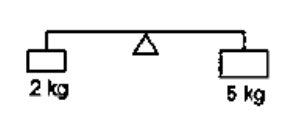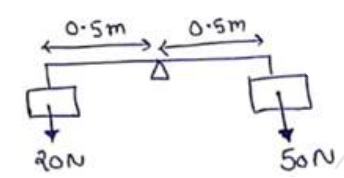# Solve the following :Question:

A light rod of length $1 \mathrm{~m}$ is pivoted at its centre and two masses of $5 \mathrm{~kg}$ ang $2 \mathrm{~kg}$ are hung from the ends as shown in figure. Find the initial angular acceleration of the rod assuming that it was horizontal in the beginning.Solution:$\tau_{\text {Net }}=50 \times 0.5-20 \times 0.5$

$\tau_{\text {Net }}=15 \mathrm{~N}-\mathrm{m}$

$\tau=I \alpha$

$15=\left[(2)(0.5)^{2}+5(0.5)^{2}\right] \alpha$

$\alpha=8.57 \sec ^{2}$